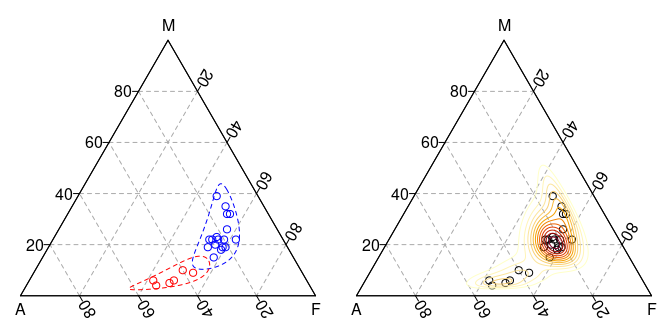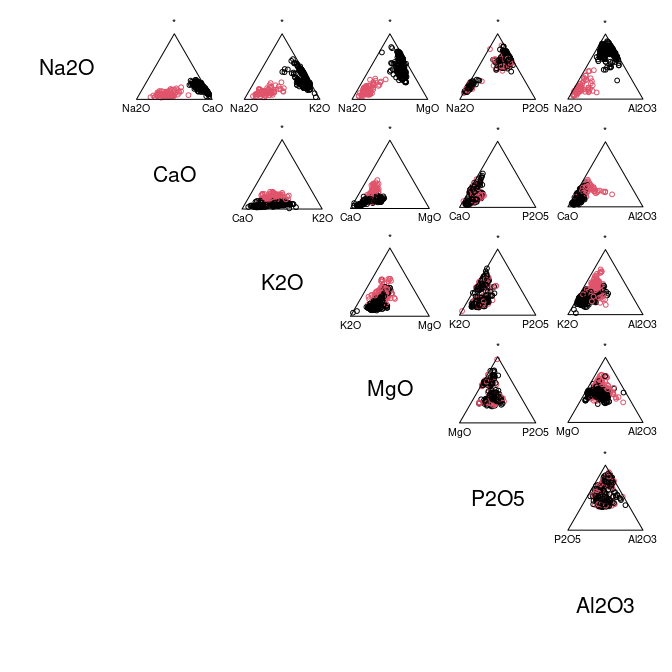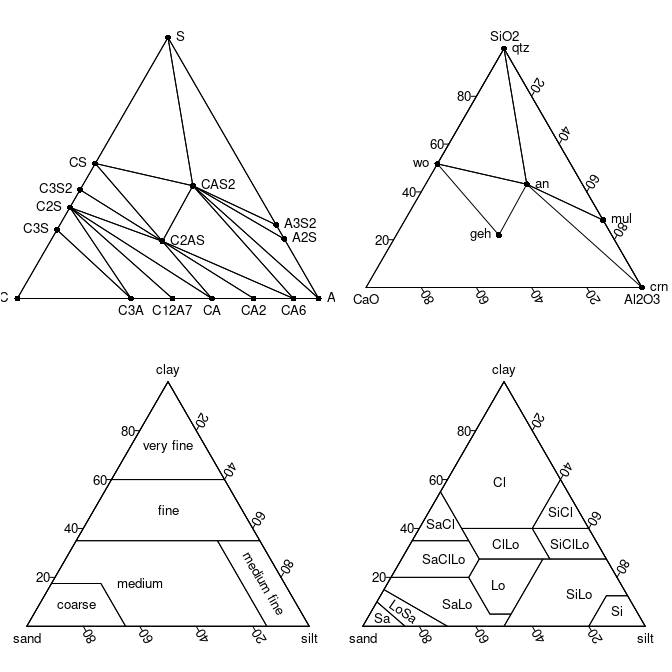## Overview

Ternary plots made simple. isopleuros allows to create ternary plot using graphics. It provides functions to display the data in the ternary space, to add or tune graphical elements and to display statistical summaries. It also includes common ternary diagrams useful for the archaeologist (e.g. soil texture charts, ceramic phase diagram).

isopleuros is a dependency-free package1 designed to be as simple as possible. If you need finer tuning or more advanced features, you should consider the Ternary or ggtern package.

To cite isopleuros in publications use:

Frerebeau N (2023). _isopleuros: Ternary Plots_. Université Bordeaux
Montaigne, Pessac, France. R package version 1.0.0,
<https://packages.tesselle.org/isopleuros/>.

Une entrée BibTeX pour les utilisateurs LaTeX est

@Manual{,
author = {Nicolas Frerebeau},
title = {{isopleuros: Ternary Plots}},
year = {2023},
organization = {Université Bordeaux Montaigne},
note = {R package version 1.0.0},
url = {https://packages.tesselle.org/isopleuros/},
}

This package is a part of the tesselle project
<https://www.tesselle.org>.

## Installation

You can install the released version of isopleuros from CRAN with:

install.packages("isopleuros")

And the development version from GitHub with:

# install.packages("remotes")
remotes::install_github("tesselle/isopleuros")

## Usage

## Load package
library(isopleuros)
## Graphical parameters
par(mfrow = c(1, 2), mar = c(0, 0, 0, 0) + 0.1)

## Set colors
col <- c("blue", "red")

## Ternary plot
## (data from Aitchison 1986)
ternary_plot(
x = lava,
panel.first = ternary_grid(),
col = col[as.factor(lava$A > 30)], ) ## Split data groups <- split(lava, f = lava$A > 30)

for (i in seq_along(groups)) {
ternary_tolerance(groups[[i]], level = 0.975, lty = 2, border = col[[i]])
}

## Density contours
ternary_plot(lava, panel.first = ternary_grid())
ternary_density(lava, n = 500, nlevels = 10)## Install extra package (if needed)
# install.packages("folio")

## Data from Barrera and Velde 1989
data("verre", package = "folio")

## Select data
coda <- verre[, c("Na2O", "CaO", "K2O", "MgO", "P2O5", "Al2O3")]

## Ternary plots with marginal compositions
ternary_pairs(coda, col = as.factor(coda\$Na2O > 5))## Graphical parameters
par(mfrow = c(2, 2), mar = c(0, 0, 0, 0) + 0.1)

## Ceramic phase diagram
ternary_plot(NULL, axes = FALSE, ann = FALSE, frame.plot = TRUE)
triangle_phase_cas(symbol = TRUE, pch = 16)

ternary_plot(NULL, xlab = "CaO", ylab = "Al2O3", zlab = "SiO2")
triangle_phase_ceramic(symbol = TRUE, pch = 16)

## HYPRES soil texture
ternary_plot(NULL, xlab = "sand", ylab = "silt", zlab = "clay")
triangle_soil_hypres()

## USDA (1951) soil texture
ternary_plot(NULL, xlab = "sand", ylab = "silt", zlab = "clay")
triangle_soil_usda(symbol = TRUE)## Contributing

Please note that the isopleuros project is released with a Contributor Code of Conduct. By contributing to this project, you agree to abide by its terms.